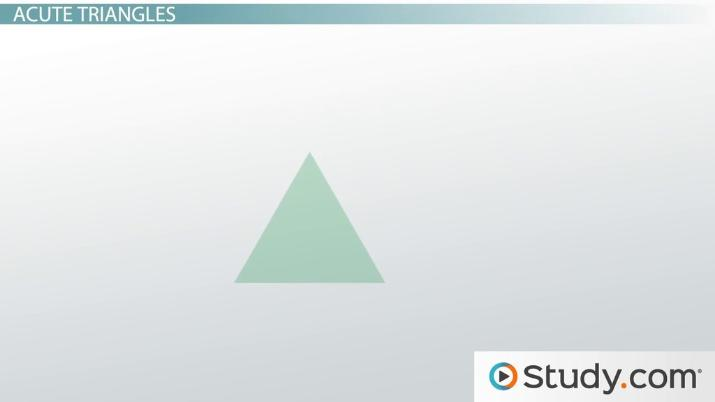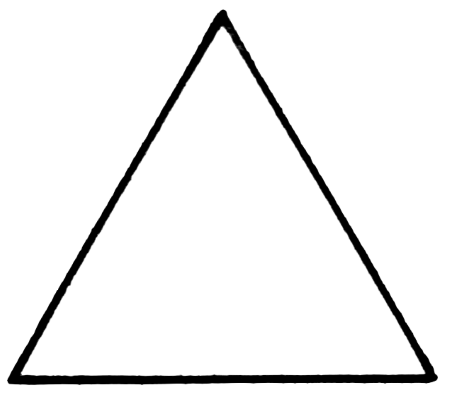# Classification of Triangles

Louise Fisher, Jeff Calareso
• Author
Louise Fisher

Louise Fisher has taught middle school students introductory physics topics for two years. She earned her bachelor's in Physics and Astronomy from the University of North Carolina at Asheville. She currently works as a physicist assistant at a cancer treatment center.

• Instructor
Jeff Calareso

Jeff teaches high school English, math and other subjects. He has a master's degree in writing and literature.

Discover the classifications of triangles and learn how to classify triangles by sides and angles. Explore the properties of scalene, isosceles, equilateral, acute, obtuse, and right triangles. Updated: 01/04/2022

Show

## How to Classify Triangles

Most people are familiar with the shape of a triangle, but what makes a triangle?

Triangles are geometrical shapes defined by having three vertices, three sides, and three interior angles that sum to 180{eq}° {/eq}. Interior angles are the angles that lie within the triangle formed by the sides. Triangles are a very common shape in math and nature, however there are many different types of triangles out there due to their broad definition. How do you classify a triangle? Tell several people to draw a triangle and they may have different results.

Since triangles just have to have three sides and three interior angles that sum to 180{eq}° {/eq}, there are quite a few different combinations of side lengths and interior angles that would form a triangle. For this reason, triangles are classified or grouped up by these side lengths and angle combinations so mathematicians can easily refer to a specific type of triangle.An error occurred trying to load this video.

Try refreshing the page, or contact customer support.

Coming up next: Interior and Exterior Angles of Triangles: Definition & Examples

### You're on a roll. Keep up the good work!

Replay
Your next lesson will play in 10 seconds
• 0:05 Classifying Triangles
• 0:40 Scalene, Isosceles &…
• 2:46 Acute Triangles
• 3:15 Obtuse Triangles
• 4:16 Right Triangle1
• 4:51 Lesson Summary
Save Save

Want to watch this again later?

Timeline
Autoplay
Autoplay
Speed Speed

## Classifying Triangles by Sides

The first way to classify triangles is by sides. Since triangles only have to have three sides to be considered a triangle, there are different side length combinations that would allow this to happen. This does not refer specifically to the unit measure of the sides, but rather the ratio of the side lengths to one another. In other words, classifying triangles by side length categorizes triangles by how many of the sides are of the same length. These triangles include scalene triangles, isosceles triangles, and equilateral triangles.

### Scalene Triangles

Scalene triangles are triangles in which none of the side lengths are the same. For this reason, they also have three different interior angle measurements. Scalene triangles do not have a line of symmetry, meaning no matter where a line is drawn through a scalene triangle, the left and right sides of the line will never mirror each other.Notice how the illustration of the scalene triangle has three different side lengths and no line of symmetry. This is what defines a scalene triangle, and for this reason it is a triangle classified by its side lengths.

### Isosceles Triangles

Another type of triangle classified by its side lengths are isosceles triangles. Isosceles triangles are triangles that have two sides of the same length. Their name is derived from the Greek words iso which means same and skelos which means leg. Isosceles triangles are very common in textile patterns due to their tessellation or ability to be repeated on a service without wasting space.### Equilateral Triangle

Perhaps one of the most common types of triangles out there are equilateral triangles. These are the triangles most children interact with when learning their basic shapes. Equilateral triangles are triangles that have three sides of equal length, hence the name equilateral.## Classifying Triangles by Angles

Similar to how triangles can be classified by sides, triangles can also be classified by their angles. All triangles have three interior angles whose angle measurements sum to 180{eq}° {/eq}. There are a few different combinations of angles that can make this happen. Recall that there are different types of angles in geometry that are categorized by their angle measurement, including acute angels, right angles, and obtuse angles. The classification of triangles by angles are based on what types of angles are present within the triangle.

### Acute Triangles

Triangles that contain three acute interior angles are classified as acute triangles. Acute angles are smaller angles that have an angle measurement of less than 90{eq}° {/eq}. Thus, acute triangles are triangles in which all three interior angles measure less than 90{eq}° {/eq}.All equilateral triangles are acute triangles due to the fact that they have three sides of equal length. Since all three sides are equal, all three interior angles must also be equal in measure. These three equal interior angles must be acute since all the interior angle measurements within a triangle must sum to 180{eq}° {/eq}, and 180{eq}° {/eq} divided three ways equals out to 60{eq}° {/eq}.

These triangles are also known as equiangular, meaning that all three angles have the same measurement. Therefore, equilateral triangles are equiangular as well as acute.

### Obtuse Triangles

Obtuse angles are larger angles with an angle measurement greater than 90{eq}° {/eq}. When one of the interior angles of a triangle are obtuse, it is classified as an obtuse triangle.Obtuse triangles can only contain one obtuse angle due to the fact that the interior angle measurements must sum to 180{eq}° {/eq}. It is not possible to have a triangle with more than one obtuse angle for this reason. This also means that obtuse triangles must also contain two acute angles.

To unlock this lesson you must be a Study.com Member.

#### What are two ways to classify triangles?

Triangles are classified by their sides and their interior angles. Classifying triangles by side would take their side lengths into consideration while classifying triangles by their angles focuses on the types of angles within the triangle.

#### How do you classify a triangle by its sides?

Classifying a triangle by its sides focuses on the side lengths of the triangle and how many of them are the same. A triangle could have three sides of unequal length (scalene), two sides of the same length (isosceles), or all three sides of equal length (equilateral).

#### What are the classifications of a triangle?

Triangles can be classified by their side lengths or their interior angles. When classifying a triangle by its sides, the number of sides of equal length are taken into account. When categorizing a triangles by its interior angles, the types of angles present is taken into account.

### Register to view this lesson

Are you a student or a teacher?